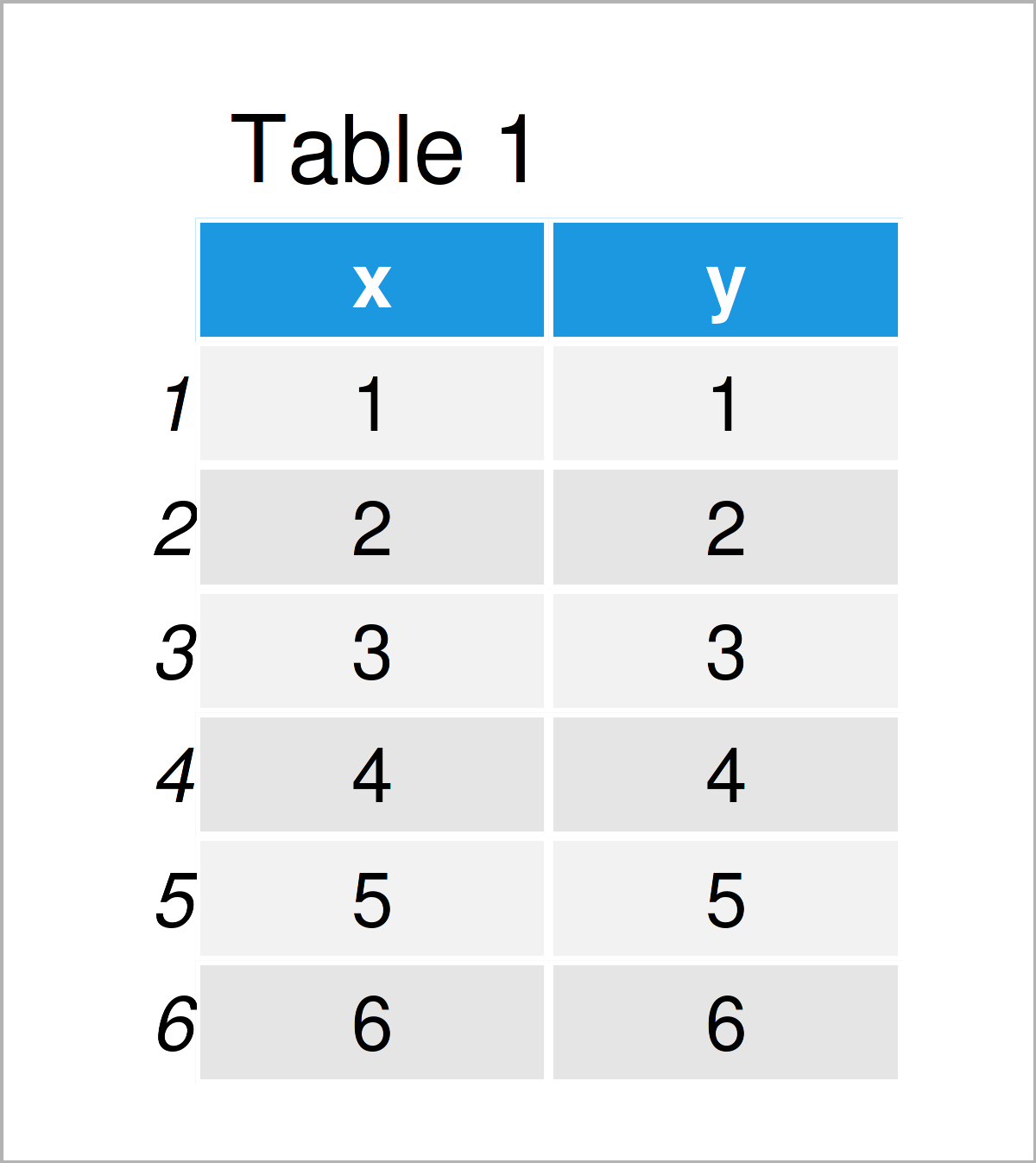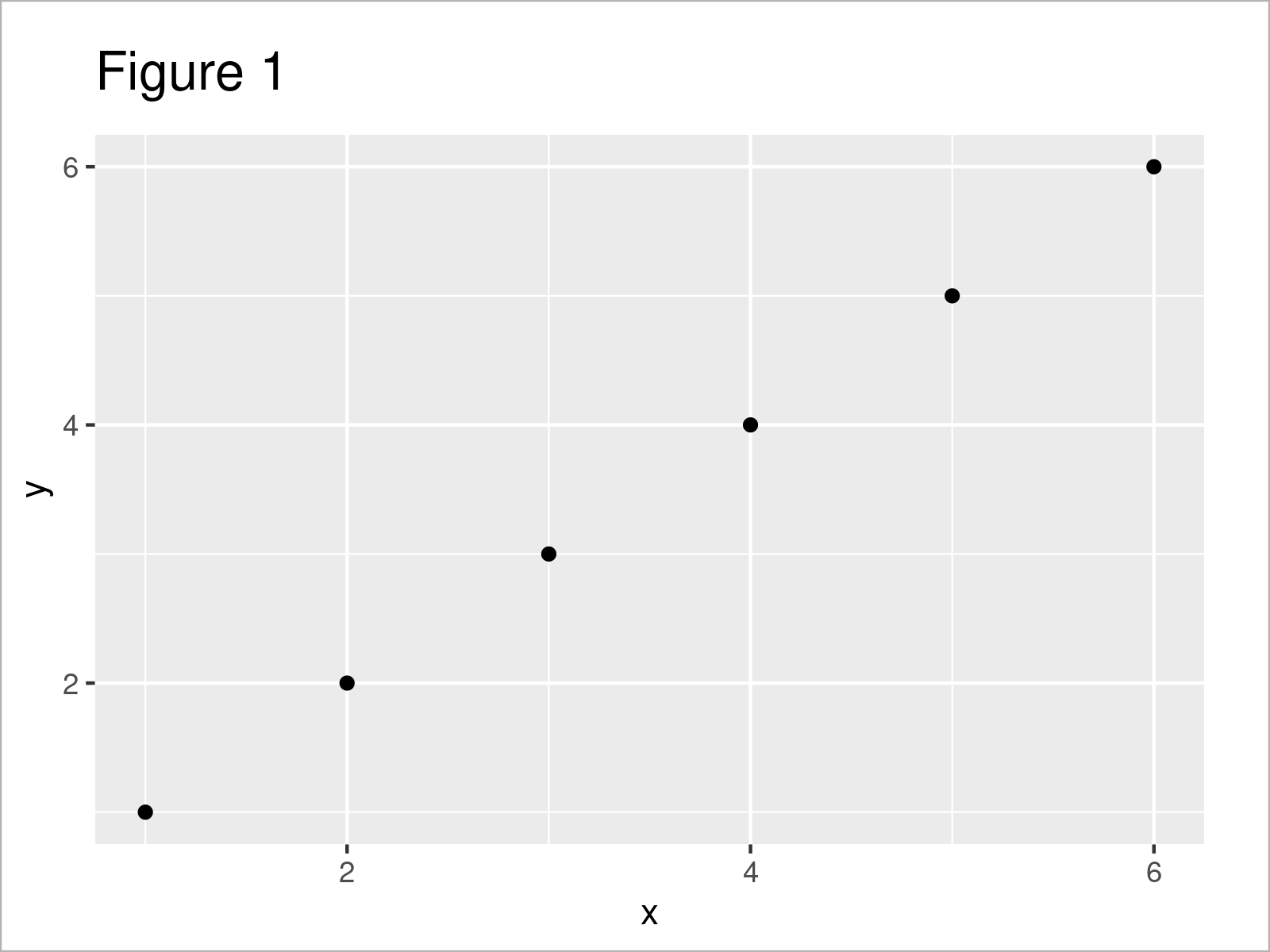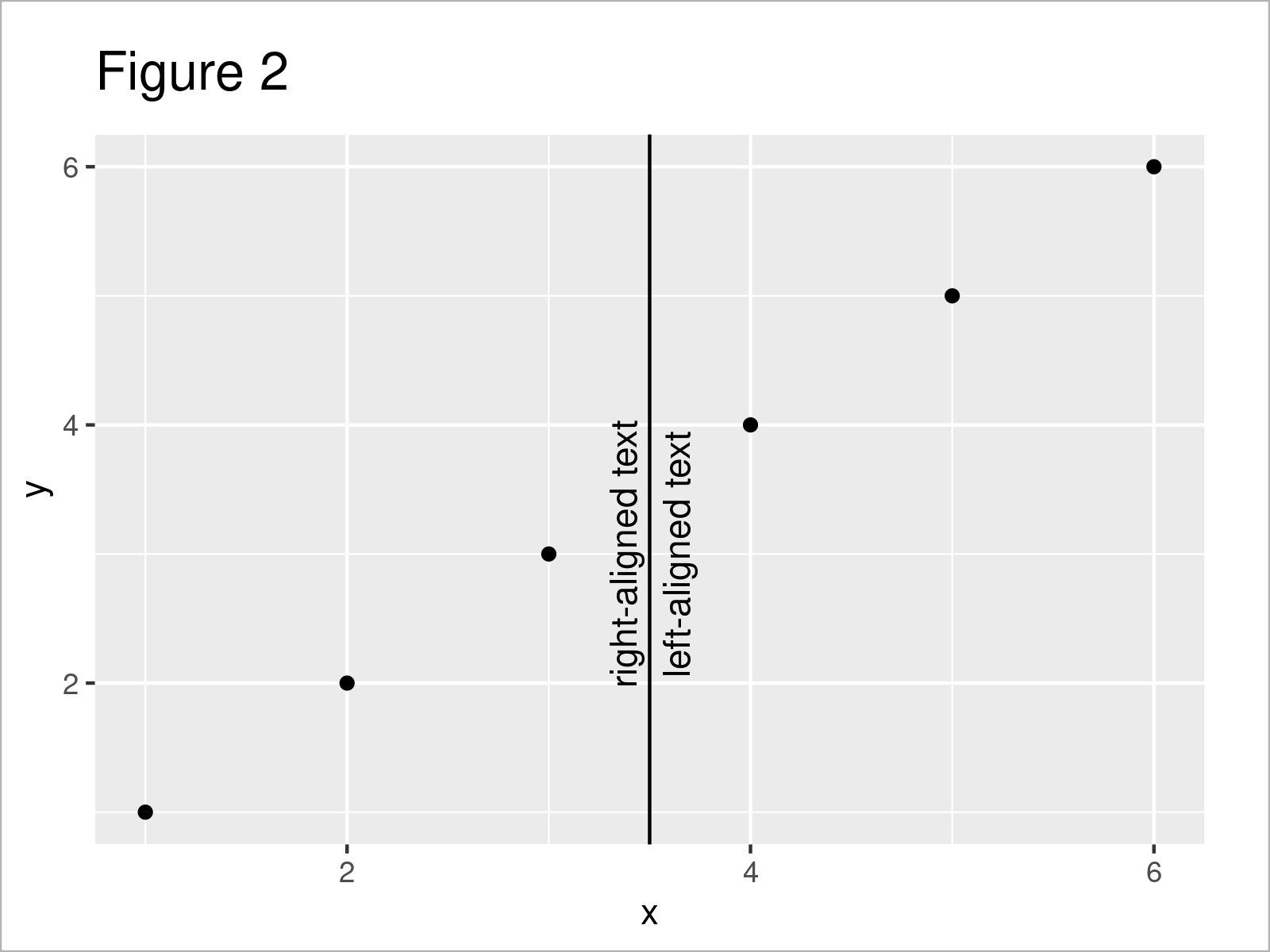# Align Text to Line in ggplot2 Plot in R (Example)

This article shows how to align text and lines in a ggplot2 plot in the R programming language.

The tutorial will consist of one example for the alignment of a text element to a line in a ggplot2 plot. To be more precise, the content of the article is structured as follows:

With that, let’s dive right into the example!

## Example Data, Packages & Basic Plot

The following data is used as basement for this R tutorial:

```data <- data.frame(x = 1:6,         # Create example data
y = 1:6)
data                                # Print example data```As you can see based on Table 1, our example data is a data frame consisting of six rows and two integer variables called “x” and “y”.

We also need to install and load the ggplot2 package, if we want to use the corresponding functions:

```install.packages("ggplot2")         # Install ggplot2 package

As a next step, we can plot our data:

```ggp <- ggplot(data, aes(x, y)) +    # ggplot2 scatterplot without line & text
geom_point()
ggp```The output of the previous R programming code is shown in Figure 1: A ggplot2 scatterplot without any line or text elements.

## Example: Add Aligned Line & Text to ggplot2 Plot Using annotate() Function

In this section, I’ll illustrate how to draw a vertical line with aligned text elements.

To achieve this, we can apply the geom_vline and annotate functions as shown in the following R code:

```ggp +                               # Draw line and text
geom_vline(xintercept = 3.5) +
annotate("text",
x = 3.5,
y = 3,
angle = 90,
label = "right-aligned text\n") +
annotate("text",
x = 3.5,
y = 3,
angle = 90,
label = "\nleft-aligned text")```After executing the previously shown R programming code the ggplot2 plot with text aligned to a vertical line shown in Figure 2 has been plotted.

As you can see in the previous code, we were able to do this based on a line break (i.e. “\n”) after / before the text element.

Note that we could apply a similar logic in case we want to draw a horizontal line with text.

## Video & Further Resources

I have recently published a video instruction on my YouTube channel, which explains the R programming codes of this tutorial. Please find the video below:

Please accept YouTube cookies to play this video. By accepting you will be accessing content from YouTube, a service provided by an external third party.If you accept this notice, your choice will be saved and the page will refresh.

Furthermore, you could have a look at the other articles on my website. I have released several other posts on related topics such as regression models, lines, dates, and ggplot2:

To summarize: In this article, I have shown how to align text elements to lines in a ggplot2 plot in the R programming language. Please tell me about it in the comments section below, if you have additional comments and/or questions.

Subscribe to the Statistics Globe Newsletter